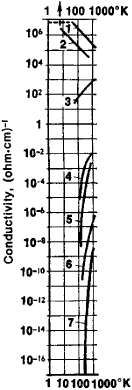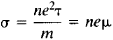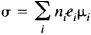# Conductivity

(redirected from conductivities)
Also found in: Dictionary, Thesaurus, Medical.

## conductivity

1. the property of transmitting heat, electricity, or sound
2.
a. a measure of the ability of a substance to conduct electricity; the reciprocal of resistivity
b. in the case of a solution, the electrolytic conductivity is the current density divided by the electric field strength, measured in siemens per metre
Collins Discovery Encyclopedia, 1st edition © HarperCollins Publishers 2005
The following article is from The Great Soviet Encyclopedia (1979). It might be outdated or ideologically biased.

## Conductivity

Electrical conductivity in a biological system is due to the presence of ions and mobile polar molecules within the system. Biological tissue consists of cells and of an intercellular space filled with an electrolyte that has a specific resistance of approximately 100 ohm centimeters. The cell contents are separated from the intercellular space by a membrane whose equivalent electrical circuit is a combination of a resistance and a capacitance in parallel. Hence the conductivity of biological tissues varies with the frequency and waveform of the current in the tissues. The specific resistance and capacitance of a cell membrane are of the order of 1 kilohm cm2 and 1 microfarad per cm2, respectively. Some tissues are capable of excitation in response to a current, in which case their conductivity is not linearly dependent on the amplitude of the current. In the absence of excitation, the current is distributed through the tissues in accordance with the impedance of the tissue components.

Since cell membranes offer comparatively high resistance to low-frequency currents (≲1 kilohertz), the major portion of the current passes through the intercellular openings. The amplitude of low-frequency currents is proportional to the volume of the intercellular space (for example, to the lumen of a blood vessel) and to the electrolytes concentrated therein. In biology and medicine, the conductivity of tissues at such low frequencies is measured in order to determine the amount of blood in various organs and to detect edema—a condition in which the intercellular space in an organ is reduced by the swollen cells. The conductivity of tissues measured at frequencies higher than 100 kilohertz is proportional to the total amount of electrolytes contained in the tissue between the electrodes, since the cell membranes in this case no longer prevent the electric current from flowing. The measurement of conductivity at such high frequencies is used in biology and medicine to record the slight changes in an organ’s volume produced by the inflow or outflow of blood. Knowledge of the conductivity of a biological system is essential not only for what it reveals about the system’s structure but also for its use in designing instruments that have biological tissues in their input and output circuits.

### REFERENCE

Cole, K. S. “Ionnaia elektroprovodnost’ nervov.” (Translated from English.) In the collection Protsessy regulirovaniia v biologii. Moscow, 1960.
Schwan, H. “Spektroskopiia biologicheskikh veshchestv v pole peremennogo toka.” In the collection Elektronika i kibernetika v biologii i medilsine. Moscow, 1963. (Translated from English.)
Ackerman, E. Biofizika. Moscow, 1964. Pages 222–27. (Translated from English.)
Cole, K. S. “Nervnyi impul’s (teoriia i eksperiment).” In the collection Teoreticheskaia i matematicheskaia biologiia. Moscow, 1968.

K. IU. BOGDANOV

## Conductivity

(also electrical conductivity or specific conductance), the ability of a body to conduct electric current under the influence of an electric field. The term “conductivity” is also applied to the physical quantity that characterizes such ability.

In contrast to insulators, or dielectrics, bodies that conduct electric current are called conductors. Conductors always contain free or quasi-free charge carriers, such as electrons and ions, whose directed motion constitutes electric current. The conductivity of most conductors—that is, of metals, semiconductors, and plasmas—is due to electrons; in plasmas, ions make a small contribution to conductivity. Ionic conductivity is characteristic of electrolytes.

Electric current I depends on the voltage V that is applied to a conductor, and the voltage determines the electric field intensity E in the conductor. For an isotropic conductor of constant cross section, E = – VIL, where L is the length of the conductor. The current density j depends on the magnitude of E at a given point and, in isotropic conductors, has the same direction as E. The relationship between j and E is given by Ohm’s law: j = σE; the factor σ is independent of E and is called conductivity. The quantity that is the reciprocal of σ is referred to as resistivity: ρ = 1/σ. For various types of conductors, the values of σ or of ρ differ substantially (see Figure 1). In the general case, the relationship between j and E is nonlinear and σ depends on E. In this case, differential conductivity σ = dj/dE is introduced. Conductivity is measured in (ohm·cm)–1 or, in the International System of Units (SI), in (ohm·m)–1.

In anisotropic media, for example, in single crystals, σ is a tensor of rank two. The conductivity for different directions in a crystal may differ, leading to different directions of E and j.Figure 1. Dependence of the conductivity σ of certain substances on absolute temperature: metals: (1) copper, (2) lead, which becomes superconducting below 7.3°K; semiconductors: (3) graphite, (4) pure germanium, (5) pure silicon; ionic conductors: (6) sodium chloride, (7) glass

Depending on their conductivity, all substances are divided into conductors, dielectrics, and semiconductors. For conductors, σ > 106 (ohm·m)–1; for dielectrics, σ < 10–8 (ohm·m)–1. The conductivity of semiconductors is intermediate between that of conductors and dielectrics. To a considerable extent, the division into conductors, dielectrics, and semiconductors is arbitrary because the conductivity of a substance may vary over a wide range when the state of the substance changes. Conductivity depends on the temperature of a substance, on the structure of the substance (for example, the existence of an aggregate state or the presence of defects), and on external influences, such as a magnetic field, illumination, or a strong electric field.

The ratio of the mean free time τ and the characteristic collision time tcol of charge carriers, τ/tcol ≪ 1, is used as a measure of the “freedom” of charge carriers in a conductor. The larger the ratio, the higher the accuracy with which particles may be considered free. The methods of the kinetic theory of gases make it possible to express σ in terms of the density n of charge carriers, the charge e and mass m of the carriers, and their mean free time:where μ, is the mobility of a particle. The mobility μ. is equal to E/vmean, where vmean is the mean velocity of the directed motion. If a current is due to charged particles of different species i, thenOwing to the low mass of electrons, the mobility of electrons is so much greater than the mobility of ions that ionic conductivity is substantial only in the case where free electrons are virtually absent. On the other hand, mass transfer under the influence of a current is associated with the motion of ions.

The nature of the dependence of conductivity on temperature T differs for various substances. For metals, the function σ(T) is governed mainly by a decrease in the mean free time of electrons as T increases; that is, an increase in temperature leads to the growth of thermal lattice vibrations, which scatter electrons, and σ is reduced. In the language of quantum mechanics, we speak of collisions between electrons and phonons. At high enough temperatures, that is, at temperatures higher than the Debye temperature θD, the conductivity of metals is inversely proportional to temperature: σ ~ 1/T. At T ≫ θD, σ ~ T–5; however, it is limited by the residual resistance of metals (seeMETALS). In semiconductors, σ increases sharply as the temperature rises, owing to an increase in the number of conduction electrons and carriers of positive charge, or holes (seeSEMICONDUCTOR). Dielectrics have an appreciable conductivity only in electric fields of very high intensity. At a certain large value of E, dielectric breakdown occurs.

When T is reduced to several degrees Kelvin, certain metals, alloys, and semiconductors experience a transition to the superconducting state, for which σ = ∞ (seeSUPERCONDUCTIVITY). When metals are melted, their conductivity remains of the same order of magnitude as in the solid state.

The conductivity of liquids is discussed in ELECTROLYTE and in FARADAY’S LAWS OF ELECTROLYSIS.

The passage of a current through a partially or completely ionized gas—that is, through a plasma—has its own specific nature (seeELECTRIC DISCHARGES IN GASES and PLASMA). For example, in a completely ionized plasma, conductivity is independent of density and increases with increasing temperature as T3/2, reaching the conductivity of good metals.

Departures from Ohm’s law in a stationary field E occur if, as E increases, the energy acquired by particles between collisions, eEl, where l is the mean free path, becomes of the same order of magnitude as or greater than kT, where k is the Boltzmann constant. In metals, the condition eElkT is difficult to satisfy. However, in semiconductors, in electrolytes, and especially in plasmas, departure effects in strong electric fields are quite substantial.

In a variable electromagnetic field, σ depends on the frequency ω and the wavelength λ of the field. In this case, we speak of temporal dispersion and spatial dispersion, which occur at ω ≳ τ–1 and λ ≲ l, respectively. The skin effect is a characteristic property of good conductors; even at ω ≪ τ–1, the current is concentrated near the surface of a conductor.

The measurement of conductivity is an important method in the study of materials, especially in the study of the purity of metals and semiconductors. In addition, the measurement of conductivity makes it possible to clarify the dynamics of charge carriers in a macroscopic body and to explain the nature of collisions between charge carriers or between carriers and other objects in the body.

The conductivity of metals and semiconductors depends substantially on magnetic field strength, especially at low temperatures (seeGALVANOMAGNETIC PHENOMENA).

M. I. KAGANOV

The Great Soviet Encyclopedia, 3rd Edition (1970-1979). © 2010 The Gale Group, Inc. All rights reserved.

## conductivity

[‚kän‚dək′tiv·əd·ē]
(electricity)
The ratio of the electric current density to the electric field in a material. Also known as electrical conductivity; specific conductance.
(geology)
McGraw-Hill Dictionary of Scientific & Technical Terms, 6E, Copyright © 2003 by The McGraw-Hill Companies, Inc.

## thermal conductivity

The rate of transfer of heat by conduction; the amount of heat per unit of time per unit area that is conducted through a slab of unit thickness of a material if the difference in temperature between opposite faces is one degree of temperature; a property of the material itself, usually represented by the letter k and called k factor.
McGraw-Hill Dictionary of Architecture and Construction. Copyright © 2003 by McGraw-Hill Companies, Inc.
References in periodicals archive ?
The model allows us to determine the thermal conductivities of the N-component system, which knows only the thermal conductivities of pure materials (A;) and volume parts ([[PHI].sub.i]) with respect to (4) :
3 and 5 that the measured thermal conductivities of the composites are higher than the predictions when the filler volume fraction is more than 20%.
The strong retention of water molecules in the system rendered them unsuitable for reducing thermal conductivities of construction elements.
The conductivities of ionic liquids at different temperatures follow Arrhenius relationship very well.
The typical approach to the estimation of thermal conductivities is to carry out measurements in the laboratory on samples.
However, leaching did not affect the conductivities of the 500 [mu]S/cm, 1000 [mu]S/cm, and 100 000 [mu]S/cm solutions, which did not change significantly.
Several models have been discussed in the literature to describe the relationship between thermal conductivity and porosity, assuming thermal conductivities of different mineral phases and fluids.
Recently, however, through the use of various filler systems, thermoplastic materials have been developed with thermal conductivities from 1 to 10 W/m[degrees]K.
As the electrode separation increases, the apparent conductivity represents a weighted average of the conductivities at increasingly greater depths.
Additionally, high surface area carbon blacks contribute higher conductivities (ref.

Site: Follow: Share:
Open / Close